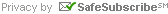# Yardage Estimators – Squares & Triangles

Yardage Estimator (Squares)

The chart below shows the number of squares that can be cut from yardages by first cutting strips and then squares. Yardage figures include the 1/4” seam allowance.

Size of square from 44” / 45” wide fabricsYardage Estimator (Triangles)

The chart below shows the number of triangles that can be cut from yardages by first cutting strips, then squares and sub-cutting the squares diagonally one way to yield half-square triangles. Yardage figures include the 1/4” seam allowance.

Size of Triangle from 44” / 45” wide fabrics* to determine the cut square size for a half square triangle, add 7/8” to the finished measurement of the triangle’s short side.

Yardage Estimator (Quarter Square & Setting Triangles)

The chart below shows the number of setting triangles that can be cut from yardages by first cutting strips, then squares and sub-cutting the squares diagonally both ways to yield quarter-square triangles. Yardage figures include the 1/4” seam allowance.

Size of Triangle from 44” / 45” wide fabrics* to determine the cut square size for a quarter square triangle, add 1 1/4” to the finished measurement of the triangle’s long side